# JP2019040403A - Semiconductor device and image recognition system - Google Patents

## Info

Publication number
JP2019040403A
JP2019040403A JP2017162057A JP2017162057A JP2019040403A JP 2019040403 A JP2019040403 A JP 2019040403A JP 2017162057 A JP2017162057 A JP 2017162057A JP 2017162057 A JP2017162057 A JP 2017162057A JP 2019040403 A JP2019040403 A JP 2019040403A
Authority
JP
Japan
Prior art keywords
circuit
input
coefficient
register
Prior art date
Legal status (The legal status is an assumption and is not a legal conclusion. Google has not performed a legal analysis and makes no representation as to the accuracy of the status listed.)
Pending
Application number
JP2017162057A
Other languages
Japanese (ja)
Inventor

Atsushi Nakamura

Rei Udagawa

Shigeru Matsuo

Original Assignee
ルネサスエレクトロニクス株式会社
Renesas Electronics Corp
Priority date (The priority date is an assumption and is not a legal conclusion. Google has not performed a legal analysis and makes no representation as to the accuracy of the date listed.)
Filing date
Publication date
Application filed by ルネサスエレクトロニクス株式会社, Renesas Electronics Corp filed Critical ルネサスエレクトロニクス株式会社
Priority to JP2017162057A priority Critical patent/JP2019040403A/en
Publication of JP2019040403A publication Critical patent/JP2019040403A/en
Application status is Pending legal-status Critical

• 239000004065 semiconductor Substances 0 abstract claims description title 31
• 238000007792 addition Methods 0 abstract claims description 49
• 230000001186 cumulative Effects 0 abstract claims description 45
• 239000000047 products Substances 0 abstract claims description 32
• 238000004364 calculation methods Methods 0 claims description 14
• 238000003860 storage Methods 0 claims description 7
• 230000000875 corresponding Effects 0 claims description 3
• 239000000872 buffers Substances 0 description 9
• 238000006011 modification Methods 0 description 7
• 230000004048 modification Effects 0 description 7
• 238000000034 methods Methods 0 description 6
• 230000003213 activating Effects 0 description 5
• 238000003384 imaging method Methods 0 description 3
• 239000011159 matrix materials Substances 0 description 2
• 230000001965 increased Effects 0 description 1
• 230000001537 neural Effects 0 description 1
• 230000003287 optical Effects 0 description 1
• 230000002829 reduced Effects 0 description 1
• 230000001603 reducing Effects 0 description 1
• 230000011218 segmentation Effects 0 description 1

## Images

•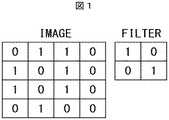•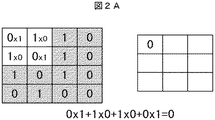••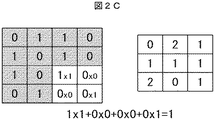••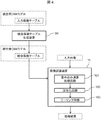••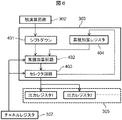•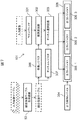•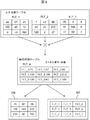••••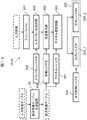•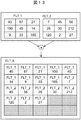•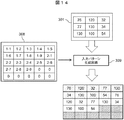## Classifications

• GPHYSICS
• G06COMPUTING; CALCULATING; COUNTING
• G06TIMAGE DATA PROCESSING OR GENERATION, IN GENERAL
• G06T1/00General purpose image data processing
• G06T1/20Processor architectures; Processor configuration, e.g. pipelining
• GPHYSICS
• G06COMPUTING; CALCULATING; COUNTING
• G06NCOMPUTER SYSTEMS BASED ON SPECIFIC COMPUTATIONAL MODELS
• G06N3/00Computer systems based on biological models
• G06N3/02Computer systems based on biological models using neural network models
• G06N3/08Learning methods
• GPHYSICS
• G06COMPUTING; CALCULATING; COUNTING
• G06FELECTRIC DIGITAL DATA PROCESSING
• G06F17/00Digital computing or data processing equipment or methods, specially adapted for specific functions
• G06F17/10Complex mathematical operations
• G06F17/15Correlation function computation including computation of convolution operations
• G06F17/153Multidimensional correlation or convolution
• GPHYSICS
• G06COMPUTING; CALCULATING; COUNTING
• G06KRECOGNITION OF DATA; PRESENTATION OF DATA; RECORD CARRIERS; HANDLING RECORD CARRIERS
• G06K9/00Methods or arrangements for reading or recognising printed or written characters or for recognising patterns, e.g. fingerprints
• G06K9/00973Hardware and software architectures for pattern recognition, e.g. modular organisation
• GPHYSICS
• G06COMPUTING; CALCULATING; COUNTING
• G06KRECOGNITION OF DATA; PRESENTATION OF DATA; RECORD CARRIERS; HANDLING RECORD CARRIERS
• G06K9/00Methods or arrangements for reading or recognising printed or written characters or for recognising patterns, e.g. fingerprints
• G06K9/36Image preprocessing, i.e. processing the image information without deciding about the identity of the image
• G06K9/46Extraction of features or characteristics of the image
• G06K9/4604Detecting partial patterns, e.g. edges or contours, or configurations, e.g. loops, corners, strokes, intersections
• G06K9/4609Detecting partial patterns, e.g. edges or contours, or configurations, e.g. loops, corners, strokes, intersections by matching or filtering
• G06K9/4619Biologically-inspired filters, e.g. receptive fields
• G06K9/4623Biologically-inspired filters, e.g. receptive fields with interaction between the responses of different filters
• G06K9/4628Integrating the filters into a hierarchical structure
• GPHYSICS
• G06COMPUTING; CALCULATING; COUNTING
• G06NCOMPUTER SYSTEMS BASED ON SPECIFIC COMPUTATIONAL MODELS
• G06N3/00Computer systems based on biological models
• G06N3/02Computer systems based on biological models using neural network models
• G06N3/04Architectures, e.g. interconnection topology
• GPHYSICS
• G06COMPUTING; CALCULATING; COUNTING
• G06NCOMPUTER SYSTEMS BASED ON SPECIFIC COMPUTATIONAL MODELS
• G06N3/00Computer systems based on biological models
• G06N3/02Computer systems based on biological models using neural network models
• G06N3/04Architectures, e.g. interconnection topology
• G06N3/0454Architectures, e.g. interconnection topology using a combination of multiple neural nets
• GPHYSICS
• G06COMPUTING; CALCULATING; COUNTING
• G06NCOMPUTER SYSTEMS BASED ON SPECIFIC COMPUTATIONAL MODELS
• G06N3/00Computer systems based on biological models
• G06N3/02Computer systems based on biological models using neural network models
• G06N3/06Physical realisation, i.e. hardware implementation of neural networks, neurons or parts of neurons
• G06N3/063Physical realisation, i.e. hardware implementation of neural networks, neurons or parts of neurons using electronic means
• GPHYSICS
• G06COMPUTING; CALCULATING; COUNTING
• G06TIMAGE DATA PROCESSING OR GENERATION, IN GENERAL
• G06T7/00Image analysis
• G06T7/0002Inspection of images, e.g. flaw detection

## Abstract

To solve the problem in which CNN requires to repeat, for the number of channels, the convolutional operation of an input image and a coefficient, and thus the computation amount is extremely voluminous.SOLUTION: A semiconductor device comprises an image recognition device including a convolutional operation processing circuit. The convolutional operation processing circuit comprises: a coefficient register in which the coefficient of an integrated coefficient table is set; a product operation circuit for computing the product of an input image and the coefficient; a channel register in which a channel number for the integrated coefficient table is set; a channel selection circuit for selecting the output destination of cumulative addition operation based on the channel number; and a plurality of output registers in which the result from the cumulative addition operation is stored. The integrated coefficient table is made by integrating a plurality of input coefficient tables and the size of the integrated coefficient table is N×N. The product operation circuit is able to compute the data in the size of N×N at a time.SELECTED DRAWING: Figure 7

## Description

The present disclosure relates to a semiconductor device, and can be applied to a semiconductor device including an image recognition device, for example.

Since the Convolutional Neural Network (CNN) won first place in the International Image Classification Contest (ILSVRC) in 2012, CNN has been used in many image recognition processes. Usefulness is recognized. In recent years, there has been an increasing demand for utilizing CNN not only for workstations with abundant resources but also for embedded devices. About CNN, it is disclosed by Unexamined-Japanese-Patent No. 2008-310700 and Unexamined-Japanese-Patent No. 2006-99707, for example.

JP 2008-310700 A Japanese Patent Laid-Open No. 2006-99707

However, the CNN needs to repeat the convolution calculation of the input image and the coefficient as many as the number of channels, and the amount of calculation is extremely large. is there.
Other problems and novel features will become apparent from the description of the specification and the accompanying drawings.

An outline of typical ones of the present disclosure will be briefly described as follows.
That is, the semiconductor device has a function of cumulative addition for each channel, and performs convolution calculations on a plurality of channels in parallel.

According to the semiconductor device, it is possible to reduce the amount of convolution calculations.

Diagram explaining the concept of convolution Diagram explaining the concept of convolution Diagram explaining the concept of convolution Diagram explaining the concept of convolution The figure which shows the structural example of an image recognition system The figure which shows the data flow of the image recognition system of FIG. The figure explaining the structural example of the convolution arithmetic processing circuit of FIG. FIG. 5 is a diagram illustrating a configuration example of the channel selection circuit in FIG. The figure explaining the structure of a 3 * 3 convolution arithmetic processing circuit Schematic diagram for explaining the operation of the integrated coefficient table generation device of FIG. Conceptual diagram for explaining the operation of the convolution operation processing circuit of FIG. Conceptual diagram for explaining the operation of the cumulative addition circuit of FIG. The figure explaining the other structural example of the convolution arithmetic processing circuit of FIG. The figure explaining the structure of a 5 * 5 convolution arithmetic processing circuit Schematic diagram for explaining the operation of the integrated coefficient table generating device of FIG. Schematic diagram for explaining the operation of the input pattern generation circuit of FIG.

First, the CNN convolution operation will be described with reference to FIGS. FIG. 1 is a diagram for explaining the concept of convolution calculation, and shows an image and a filter. 2A to 2C are diagrams for explaining the concept of the convolution operation, and are diagrams illustrating the product-sum operation of the pixel value of the image and the coefficient of the filter.

In order to make the explanation easy to understand, if the image (IMAGE) is simplified and represented by a number, it can be considered as a matrix of height × width. As shown on the left in FIG. 1, the input image is represented by binary values of 4 × 4, for example. As shown on the right side of FIG. 1, a 2 × 2 filter (FILTER) is applied to such a matrix to perform a convolution operation.

As shown in FIG. 2A, a filter is superimposed on the upper left portion of the image, and values at the same position are multiplied. The result of multiplication is added and output. As shown in FIG. 2B, the values are similarly multiplied by shifting the filter horizontally by one pixel. The result of multiplication is added and output. Hereinafter, as shown in FIG. 2C, the input image is multiplied and added element by element in the filter unit to the lower right.

When performing the convolution operation, it is necessary to repeat the convolution operation of the input image and the filter coefficient by the number of channels (the number of filters).

In the embodiment, a function of cumulative addition for each channel is provided, and a plurality of channels are convolved in parallel. Since the number of channels equivalent to the number of blocks that can be cumulatively added for each channel can be simultaneously convolved, the processing time can be shortened.

By combining the convolution operation processing circuit and the integrated coefficient table, convolution operations for a plurality of channels can be performed at one time. CNN can be realized with a small number of product-sum operations without useless product-sum operations. By using the input pattern generation circuit, any convolution operation can be performed without depending on the filter size.

Hereinafter, examples and modifications will be described with reference to the drawings. However, in the following description, the same components may be denoted by the same reference numerals and repeated description may be omitted.

FIG. 3 is a block diagram illustrating a configuration example of the image recognition system. FIG. 4 is a diagram showing a data flow of the image recognition system of FIG.

As shown in FIG. 3, the image recognition system 1 includes a semiconductor device 10 and a semiconductor memory device (MEMORY) 20. The semiconductor device 10 includes an image recognition unit (IRU) 11, a central processing unit (CPU) 12, a control circuit (MC) 13 of the semiconductor storage device 20, and a bus 14 for connecting them in one semiconductor chip. Prepare. The semiconductor memory device 20 is, for example, a DDR-SDRAM.

As shown in FIG. 4, the integrated coefficient table generating device 50 integrates input coefficient tables included in the pre-integrated CNN model stored in the storage device, generates an integrated coefficient table of the post-integrated CNN model, and the semiconductor storage device 20. The image recognition device 11 of the image recognition system 1 processes the input image stored in the semiconductor storage device 20 based on the integrated coefficient table, and stores the processing result in the semiconductor storage device 20.

The input image is an image acquired by an imaging device (not shown) having an optical system (lens) and an imaging device, and is the whole or a part of the image acquired by the imaging device. The input image is stored in the semiconductor memory device 20.

The image recognition apparatus 11 calculates using the integration coefficient table of the post-integration CNN model and the input image, and outputs the processing result. The image recognition device 11 is a device that performs necessary calculations in the post-integration CNN model.

The processing result is an output of the image recognition device 11 and is, for example, an input image classification result, a segmentation result, or a specific object detection result. The processing result is stored in the semiconductor memory device 20.

The pre-integration CNN model refers to a general CNN model. For example, AlexNet, VGGNet, SegNet, R-CNN. The input coefficient table is a coefficient used for the convolution operation among the coefficients included in the pre-combination CNN model.

The integrated coefficient table generating apparatus 50 is an apparatus that analyzes an input coefficient table and generates an integrated coefficient table that can be processed with fewer convolution operations, such as a storage device that stores a central processing unit, a program of the central processing unit, and the like. Consists of.

The post-integration CNN model is a model in which the number of convolution operations of the pre-integration CNN model is reduced. The integrated coefficient table is an output of the integrated coefficient table generating device 50.

The image recognition apparatus 11 includes a convolution operation processing circuit 101, an activation circuit 102, and a pooling circuit 103, and can cumulatively add product operation results for each channel. The convolution operation processing circuit 101 adds the result of the convolution operation for each channel number corresponding to each coefficient. The activation circuit 102 and the pooling circuit 103 are circuits that perform activation processing and pooling processing, respectively. For example, a well-known logistic sigmoid function or ReLU function (Rectified Linear Units) is used for the activation process. The pooling process is a method of reducing a large image while leaving important information, for example, dividing the image into small windows and outputting the maximum value of data input from each divided window. A function or a known average pooling function that outputs an average value of input data is used.

FIG. 5 is a block diagram for explaining a configuration example of a convolution operation processing circuit. FIG. 6 is a block diagram illustrating a configuration example of the channel selection circuit of FIG.

The integrated coefficient table is a table in which coefficients used in the convolution calculation are stored. A channel number is added to the coefficient. The integrated coefficient table generation device 50 generates an integrated coefficient table from a plurality of input coefficient tables.

The convolution operation processing circuit 101 outputs a result of convolution operations for a plurality of times in one convolution operation. The convolution operation processing circuit 101 includes a line buffer 301, a product operation circuit 302, a channel selection circuit 303, an output control register 304, and an output register 305 (first output register 305_1, second output register 305_2,... N-th output register 305_N), a coefficient register 306, and a channel register 307. The line buffer 301 acquires input image data for the designated number of lines from the semiconductor memory device 20. The product calculation circuit 302 calculates the product of the coefficient register 306 and the input image from the line buffer 301. The channel selection circuit 303 adds the channel number registered in the channel register 307 to the product operation result, and cumulatively adds the product operation result to an appropriate output register 305 according to the channel number according to the output control register 304 and outputs the result. . The output control register 304 associates channel numbers with output register numbers.

As shown in FIG. 6, the channel selection circuit 303 includes a shift-down circuit 401, a cumulative addition circuit 402, a selector circuit 403, and a cumulative addition register 404. The shift down circuit 401 shifts down the product operation result by the designated number of bits and outputs the result. The cumulative addition circuit 402 adds the results output from the cumulative addition register 404 and the shift down circuit 401 for each channel number and outputs the result. The selector circuit 403 outputs the output of the cumulative addition circuit 402 to the designated output register 305.

The output control register 304, the coefficient register 306, and the channel register 307 are set by the CPU 12.

An example in which the integrated coefficient table generation device 50 generates one type of integrated coefficient table from three types of input coefficient tables and performs a convolution operation using the integrated coefficient table will be described with reference to FIGS. FIG. 7 is a block diagram showing a configuration of a 3 × 3 convolution operation processing circuit. FIG. 8 is a schematic diagram for explaining the operation of the integrated coefficient table generating apparatus of FIG. 7, and shows a case where one integrated coefficient table is generated from the input coefficient tables for three channels. FIG. 9 is a conceptual diagram illustrating the operation of the convolution operation processing circuit of FIG. FIG. 10 is a conceptual diagram for explaining the operation of the cumulative addition circuit of FIG.

The convolution operation processing circuit in FIG. 7 uses a 3 × 3 convolution operation circuit to execute 3 × 3 convolution operations for three channels at a time.

Here, the coefficient of the coefficient table used for convolution is w i, j, chanel , the table is C l, w, channel ,

And Here, i and j are integers, channel is a channel number, l is a table width, and w is a table height. The coefficients of the integrated coefficient table

Represented by However, MAX (...) Is a function that returns the maximum value among the arguments.

Also, let l i, j, FLT_A be a character string representing the type of table used for the integration coefficient table,

And However, CHANNEL (...) Is a function representing the channel number that returned the maximum value among the arguments. At this time, C 3, 3, FLT_A of the integration coefficient table

It expresses.

FIG. 8 schematically shows how the integrated coefficient table is generated when l = 3 and w = 3. The integrated coefficient table generation device 50 processes three types of input coefficient tables (FLT_1, FLT_2, and FLT_3) and outputs an integrated coefficient table (FLT_A). In the input coefficient tables (FLT_1, FLT_2, and FLT_3), the coefficient having the largest coefficient is output to the integrated coefficient table, and the coefficients are shaded. The upper left is “75” of FLT — 3, the upper is “87” of FLT — 1, and the upper right is “56” of FLT — 2. In the middle left, FLT_1 is “100”, in the middle is “144” in FLT — 3, and in the middle right is “212” in FLT_2. The lower left is “120” of FLT_2, the lower middle is “60” of FLT — 3, and the lower right is “165” of FLT_1. The shaded portions of the input coefficient tables (FLT_1, FLT_2, FLT_3) are arranged in the integrated coefficient table (FLT_A) obtained by integrating these. The integrated coefficient table (FLT_A) stores channel numbers in addition to coefficients.

As shown in FIG. 8, the convolution operation processing circuit 101 reads a coefficient and a channel number from the integrated coefficient table, and sets values in the coefficient register 306 and the channel register 307, respectively.

As shown in FIG. 9, the product calculation circuit 302 calculates the product of the value set in the coefficient register 306 and the value set in the line buffer 301 at a time by a 3 × 3 product calculation circuit. The channel selection circuit 303 sets the output of the cumulative addition circuit 402 in the output register 305 based on the value (channel number) set in the channel register 307. The output control register 304 sets the correspondence between the output register 305 and the channel register 307. In this example, the first output register 305_1 corresponds to FLT_1, the second output register 305_2 corresponds to FLT_2, and the third output register 305_3 corresponds to FLT_3. The convolution operation processing circuit 101 outputs the result of executing the convolution operation using the coefficients of FLT_1, FLT_2, and FLT_3 to the first output register 305_1, the second output register 305_2, and the third output register 305_3, respectively.
As shown in FIG. 10, the cumulative addition circuit 402 inputs the calculation result of the product calculation circuit 302 and the content of the cumulative addition register 404 based on the channel number of the channel register 307 for each channel, and adds the channels in parallel. Thereby, the cumulative addition result for each channel is obtained together. The convolution operation processing circuit 101 does not cumulatively add the results of the product operation, but cumulatively adds them separately according to channel numbers.

In the specific example, the 3 × 3 convolution operation circuit is used to execute the 3 × 3 convolution operation for 3 channels at a time. However, the embodiment is not limited to this, and the embodiment is not limited to N × N convolution. It is possible to execute N × N convolution calculations for N channels at a time using an arithmetic circuit.

<Modification>
Hereinafter, typical modifications will be illustrated. In the following description of the modified examples, the same reference numerals as those in the above-described embodiments can be used for portions having the same configurations and functions as those described in the above-described embodiments. And about description of this part, the description in the above-mentioned Example shall be used suitably in the range which is not technically consistent. In addition, a part of the above-described embodiments and all or a part of the modified examples can be appropriately combined in a range not technically inconsistent.

An example of performing a convolution operation regardless of the arrangement of input images will be described with reference to FIG. FIG. 11 is a block diagram for explaining another configuration example of the convolution operation processing circuit.

The convolution operation processing circuit 101A further includes an input pattern register 308 and an input pattern generation circuit 309 in addition to the configuration of the convolution operation processing circuit 101 of the embodiment. The input pattern register 308 is a register for setting how the pixel value set in the line buffer 301 is output from the input pattern generation circuit 309. The input pattern generation circuit 309 is a circuit that receives the line buffer 301 and generates an output according to the input pattern register 308. The input pattern register 308 is set by the CPU 12.

Next, an example in which the integrated coefficient table generation device 50 generates one type of integrated coefficient table from two types of input coefficient tables and performs a convolution operation using the integrated coefficient table will be described with reference to FIGS. . FIG. 12 is a diagram showing a configuration of a convolution operation processing circuit. FIG. 13 is a schematic diagram for explaining the operation of the integrated coefficient table generating apparatus of FIG. 12, and shows a case where one integrated coefficient table is generated from the input coefficient tables for two channels. FIG. 14 is a schematic diagram for explaining the operation of the input pattern generation circuit of FIG. 12, and shows a case where a 3 × 3 input image is generated as a 5 × 5 input image for two channels.

The convolution operation processing circuit 101A shown in FIG. 12 uses a 5 × 5 convolution operation circuit to execute 2 × 3 × 3 convolution operations at a time.

If the input coefficient table is C 3,3, FLT_1 , C 3,3, FLT_2 , and the integrated coefficient table is C 5,5, FLT_A ,

It expresses.

Also, I i, j is the pixel value (gray value) of the input image of i rows and j columns, and the output of the input pattern generation circuit 309 is A 5,5 ,

And

FIG. 13 schematically shows how the integrated coefficient table is generated when l = 3 and w = 3. The integrated coefficient table generating device 50 processes two types of input coefficient tables (FLT_1, FLT_2) and outputs an integrated coefficient table (FLT_A). The coefficients of the 3 × 3 input coefficient tables (FLT_1, FLT_2) are output to the 5 × 5 integrated coefficient table.

As shown in FIG. 13, coefficients “40”, “87”, “21” in the first row of FLT — 1 and coefficients “100”, “45” in the second row are arranged from left to right in the first row of FLT_A. . From left to right of the second row of FLT_A, the coefficient “14” at the right end of the second row of FLT_1, the coefficients “9”, “33”, “165” of the third row, and the coefficient “7” of the left end of the first row of FLT_2 Is arranged. From the left to the right of the third row of FLT_A, the middle coefficient “45”, the rightmost coefficient “56” of the first row of FLT_2, and the coefficients “25”, “30”, and “212” of the second row are arranged. The coefficients “120”, “2”, and “27” in the third row of FLT_2 are arranged from left to right in the fourth row of FLT_A. Coefficients are not arranged in the two right places of the fourth row of FLT_A and the fifth row of FLT_A, and are blank. The integrated coefficient table (FLT_A) stores channel numbers in addition to coefficients.

As shown in FIG. 14, the input pattern generation circuit 309 repeatedly outputs 3 × 3 pixels acquired from the line buffer 301 based on the 5 × 5 input pattern of the input pattern register 308. The input patterns are the first 3 × 3 pixels (1-1, 1-2,..., 1-9) and the second 3 × 3 pixels (2-1, 2-2,. 2-9) defines the order of arrangement.

As shown in FIG. 14, the pixel values “76”, “120”, “32” of the first row of the input image from the left to the right of the first row of the output of the input pattern generation circuit 309, and the pixel values “77” of the second row. ”And“ 130 ”are arranged. From the left to the right of the second row, the pixel value “34” at the right end of the second row of the input image, the pixel values “130”, “100”, “54” of the third row, the first row of the second input image The pixel value “78” at the left end is arranged. From the left to the right of the third row, the middle pixel value “120”, the rightmost pixel value “32”, and the second row pixel values “77”, “130”, and “34” of the first row are arranged. The The pixel values “130”, “100”, and “54” in the third row of the input image are arranged from the left to the right in the fourth row. Pixels are not arranged in the two right places of the fourth row and the fifth row, and are blank. As a result, the arrangement of the output pixels of the input pattern generation circuit 309 is the same as the arrangement of the coefficients in the integrated coefficient table (FLT_A).

By adding the input pattern register 308 and the input pattern generation circuit 309, the pixels stored in the line buffer 301 can be read out in an arbitrary form.

Similar to the embodiment, the convolution operation processing circuit 101A reads the coefficient and the channel number from the integrated coefficient table, and sets values in the coefficient register 306 and the channel register 307, respectively.

Similar to the embodiment, the product calculation circuit 302 calculates the product of the value set in the coefficient register 306 and the value generated by the input pattern generation circuit 309 at once by a 5 × 5 product calculation circuit. The channel selection circuit 303 sets the output of the cumulative addition circuit 402 in the output register 305 based on the value (channel number) set in the channel register 307. The output control register 304 sets the correspondence between the output register 305 and the channel register 307. In this example, the first output register 305_1 corresponds to FLT_1, and the second output register 305_2 corresponds to FLT_2. The convolution operation processing circuit 101A outputs the result of executing the convolution operation using the coefficients of FLT_1 and FLT_2 to the first output register 305_1 and the second output register 305_2, respectively.
Similar to the embodiment, the cumulative addition circuit 402 inputs the calculation result of the product calculation circuit and the content of the cumulative addition register for each channel based on the channel number of the channel register 307, and adds the channels in parallel. Thereby, the cumulative addition result for each channel is obtained together.

In the modification, an arbitrary convolution operation can be performed by using the input pattern register 308 and the integrated coefficient table generating device 50 in combination. For this reason, even if a 5 × 5 convolution operation circuit is used, 3 × 3 or 1 × 1 convolution operation can be efficiently performed.

In the specific example, the example in which the 5 × 5 convolution operation circuit is used to execute 2 × 3 × 3 convolution operations at one time has been described. Using the arithmetic circuit, it is possible to execute L times of M × M convolution operations at one time. Here, M <N and L = INT (N 2 / M 2 ) ≧ 2.

As mentioned above, the invention made by the present inventor has been specifically described based on the embodiments, examples, and modifications. However, the present invention is not limited to the above-described embodiments, examples, and modifications, and various modifications can be made. It goes without saying that it is possible.

DESCRIPTION OF SYMBOLS 1 ... Image recognition system 10 ... Semiconductor device 11 ... Image recognition apparatus 101 ... Convolution operation processing circuit 301 ... Line buffer 302 ... Product operation circuit 303 ... Channel selection circuit 401- ..Shift-down circuit 402... Cumulative addition circuit 403... Selector circuit 404... Cumulative addition register 304... Output control circuit 305. 308 ... Input pattern register 309 ... Input pattern generation circuit 102 ... Activation circuit 103 ... Pooling circuit 12 ... Central processing unit 13 ... Control circuit 14 ... Bus 20 ... Semiconductor memory device 50... Integrated coefficient table generation device

## Claims (18)

1. An image recognition device having a convolution operation processing circuit is provided,
The convolution processing circuit is
A coefficient register in which the coefficients of the integrated coefficient table are set;
A product operation circuit for obtaining a product of the input image and the coefficient;
A channel register in which a channel number of the integration coefficient table is set;
A channel selection circuit that selects an output destination of the cumulative addition operation based on the channel number;
A plurality of output registers for storing the result of the cumulative addition operation;
With
The integrated coefficient table is obtained by integrating a plurality of input coefficient tables, and the integrated coefficient table has a size of N × N,
The product operation circuit is a semiconductor device capable of calculating N × N data at a time.
2. In claim 1,
The channel selection circuit includes:
A cumulative addition circuit for adding the product for each channel number;
A cumulative addition register for holding the output of the cumulative addition circuit for each channel number;
With
The cumulative addition circuit is a semiconductor device that performs addition for each channel number in parallel.
3. In claim 2,
The channel selection circuit further includes a selector that outputs an output of the cumulative addition circuit to any one of the plurality of output registers based on the channel number.
4. In claim 1,
Each element of the integrated coefficient table is a semiconductor device in which a coefficient having the largest value is stored in each corresponding element of the plurality of input coefficient tables.
5. In claim 1,
The number of the plurality of input coefficient tables is N, and each of the plurality of input coefficient tables is a size of N × N.
6. The input pattern register according to claim 1, further comprising:
An input pattern generation circuit for changing the arrangement of the input image based on the contents of the input pattern register;
With
The integrated coefficient table is obtained by integrating L input coefficient tables, and the integrated coefficient table has a size of N × N, and each of the L input coefficient tables has a size of M × M. , M is a value smaller than N,
The product operation circuit is a semiconductor device capable of calculating N × N data at a time.
7. In claim 6,
The channel selection circuit includes:
A cumulative addition circuit for adding the product for each channel number;
A cumulative addition register for holding the output of the cumulative addition circuit for each channel number;
With
The cumulative addition circuit is a semiconductor device that performs addition for each channel number in parallel.
8. In claim 7,
The channel selection circuit further includes a selector that outputs an output of the cumulative addition circuit to any one of the plurality of output registers based on the channel number.
9. In claim 6,
The input pattern generation circuit is a semiconductor device that repeats the L number of M × M input images into an N × N input image.
10. A semiconductor device;
A semiconductor memory device;
With
The semiconductor device includes:
A coefficient register in which coefficients of the integrated coefficient table are set; a product operation circuit for obtaining a product of the input image read from the semiconductor storage device and the coefficients; a channel register in which channel numbers of the integrated coefficient table are set; A convolution operation processing circuit comprising: a channel selection circuit that selects an output destination of the cumulative addition operation based on the channel number; and a plurality of output registers that store the result of the cumulative addition operation;
A pooling circuit;
With
The product operation circuit can calculate N × N data at a time,
The integrated coefficient table is an image recognition system in which a plurality of input coefficient tables are integrated, and the integrated coefficient table has a size of N × N.
11. In claim 10,
The channel selection circuit includes:
A cumulative addition circuit for adding the product for each channel number;
A cumulative addition register for holding the output of the cumulative addition circuit for each channel number;
With
The cumulative addition circuit is an image recognition system that performs addition for each channel number in parallel.
12. In claim 11,
The channel selection circuit further includes a selector that outputs an output of the cumulative addition circuit to any one of the plurality of output registers based on the channel number.
13. In claim 10,
The image recognition system in which each element of the integrated coefficient table stores a coefficient having the largest value in each corresponding element of the plurality of input coefficient tables.
14. In claim 10,
The number of the plurality of input coefficient tables is N, and each of the plurality of input coefficient tables has a size of N × N.
15. In claim 10,
The semiconductor device further includes:
An input pattern register;
An input pattern generation circuit for changing the arrangement of the input image based on the contents of the input pattern register;
With
The integrated coefficient table is obtained by integrating L input coefficient tables, and the integrated coefficient table has a size of N × N, and each of the L input coefficient tables has a size of M × M. , M is a value smaller than N,
The product calculation circuit is an image recognition system capable of calculating N × N data at a time.
16. In claim 15,
The channel selection circuit includes:
A cumulative addition circuit for adding the product for each channel number;
A cumulative addition register for holding the output of the cumulative addition circuit for each channel number;
With
The cumulative addition circuit is an image recognition system that performs addition for each channel number in parallel.
17. In claim 16,
The channel selection circuit further includes a selector that outputs an output of the cumulative addition circuit to any one of the plurality of output registers based on the channel number.
18. In claim 15,
The input pattern generation circuit is an image recognition system that repeats the L number of M × M input images into an N × N input image.
JP2017162057A 2017-08-25 2017-08-25 Semiconductor device and image recognition system Pending JP2019040403A (en)

## Priority Applications (1)

Application Number Priority Date Filing Date Title
JP2017162057A JP2019040403A (en) 2017-08-25 2017-08-25 Semiconductor device and image recognition system

## Applications Claiming Priority (5)

Application Number Priority Date Filing Date Title
JP2017162057A JP2019040403A (en) 2017-08-25 2017-08-25 Semiconductor device and image recognition system
US16/035,010 US20190065947A1 (en) 2017-08-25 2018-07-13 Semiconductor device and image recognition system
EP18185295.5A EP3447682A1 (en) 2017-08-25 2018-07-24 Semiconductor device and image recognition system
TW107126993A TW201921287A (en) 2017-08-25 2018-08-03 Semiconductor device and image recognition system
CN201810938920.6A CN109427035A (en) 2017-08-25 2018-08-17 Semiconductor equipment and image identification system

## Publications (1)

Publication Number Publication Date
JP2019040403A true JP2019040403A (en) 2019-03-14

# Family

## Family Applications (1)

Application Number Title Priority Date Filing Date
JP2017162057A Pending JP2019040403A (en) 2017-08-25 2017-08-25 Semiconductor device and image recognition system

## Country Status (5)

US (1) US20190065947A1 (en)
EP (1) EP3447682A1 (en)
JP (1) JP2019040403A (en)
CN (1) CN109427035A (en)
TW (1) TW201921287A (en)

## Family Cites Families (5)

* Cited by examiner, † Cited by third party
Publication number Priority date Publication date Assignee Title
JP5184824B2 (en) 2007-06-15 2013-04-17 キヤノン株式会社 Arithmetic processing apparatus and method
JP5376920B2 (en) * 2008-12-04 2013-12-25 キヤノン株式会社 Convolution operation circuit, hierarchical convolution operation circuit, and object recognition device
JP6365258B2 (en) 2014-11-19 2018-08-01 株式会社デンソー Arithmetic processing unit
US9582726B2 (en) * 2015-06-24 2017-02-28 Qualcomm Incorporated Systems and methods for image processing in a deep convolution network
JP2017079017A (en) * 2015-10-21 2017-04-27 キヤノン株式会社 Convolution arithmetic apparatus

## Also Published As

Publication number Publication date
US20190065947A1 (en) 2019-02-28
TW201921287A (en) 2019-06-01
EP3447682A1 (en) 2019-02-27
CN109427035A (en) 2019-03-05

## Similar Documents

Publication Publication Date Title
EP0448367B1 (en) High speed digital parallel multiplier
US7574466B2 (en) Method for finding global extrema of a set of shorts distributed across an array of parallel processing elements
KR100415417B1 (en) Image-processing processor
JP3902741B2 (en) Semiconductor integrated circuit device
Wilkes The best way to design an automatic calculating machine
US4739474A (en) Geometric-arithmetic parallel processor
US9135553B2 (en) Convolution operation circuit and object recognition apparatus
US20180260709A1 (en) Calculating device and method for a sparsely connected artificial neural network
JP2007179563A (en) Method of optimizing graphics processing
US5151953A (en) Single chip 2-D convolver
EP1577789A2 (en) Reconfigurable circuit with connection unit
US7171535B2 (en) Serial operation pipeline, arithmetic device, arithmetic-logic circuit and operation method using the serial operation pipeline
JP4288461B2 (en) Symmetric image filter processing apparatus, program, and method
CA2215598C (en) Fpga-based processor
Uhr Parallel computer vision
GB2045488A (en) Programmable sequential logic circuit devices
US6404439B1 (en) SIMD control parallel processor with simplified configuration
JP3228927B2 (en) Processor element, processing unit, processor, and processing method
Zhang et al. A programmable vision chip based on multiple levels of parallel processors
US4791677A (en) Image signal processor
Wang et al. A fast implementation of adaptive histogram equalization
US20050286794A1 (en) Gaussian blur approximation suitable for GPU
Fife et al. Improved census transforms for resource-optimized stereo vision
US4783649A (en) VLSI graphics display image buffer using logic enhanced pixel memory cells
DE3721074A1 (en) Circuit arrangement for the displacement vector search in the digital image analysis